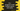# C++ program to read student names and marks# Introduction :

In this C++ tutorial, we will learn how to read name and marks for a list of students using structure. We will create one structure that can hold the name and marks for one student and we will create one array of that structure to hold the names and marks of a list of students.

This program will take all inputs from the user. We are explaining everything at the bottom of this post. Try to run this program with different inputs.

## C++ program :

``````#include <iostream>
using namespace std;

// 1
struct student
{
string name;
int totalSubjects;
int marks;
};

// 2
void printStudentDetails(student students[], int totalStudents)
{
// 3
for (int i = 0; i < totalStudents; i++)
{
cout << "Student name : " << students[i].name << endl;
cout << "Marks :" << endl;
for (int j = 0; j < students[i].totalSubjects; j++)
{
cout << students[i].marks[j] << endl;
}
}
}
int main()
{
// 4
int totalStudents;

cout << "Enter total number of students : ";

cin >> totalStudents;

// 5
if (totalStudents <= 0)
{
cout << "Please enter a valid number" << endl;
return -1;
}

student studentArray[totalStudents];

// 6
for (int i = 0; i < totalStudents; i++)
{
// 7
cout << "Enter the name of student " << (i + 1) << " : " < < endl;
cin >> studentArray[i].name;

cout << "Enter total number of subjects : ";
cin >> studentArray[i].totalSubjects;

// 8
if (studentArray[i].totalSubjects > 20 || studentArray[i].totalSubjects < = 0)
{
cout << "Please enter a valid number" << endl;
return -1;
}

// 9
for (int j = 0; j < studentArray[i].totalSubjects; j++)
{
cout << "Enter marks for subject " << (j + 1) << " : " << endl;
cin >> studentArray[i].marks[j];
}
}
// 10
printStudentDetails(studentArray, totalStudents);
}``````

### Explanation :

The commented numbers in the above program denote the step numbers below :

1. student is a structure. We will create one array of this structure type to hold the information of students. It has three properties : name to hold the name of the student, totalSubjects to hold the total number of subjects for the student and marks to hold the marks for each subjects.
2. printStudentDetails function prints the details of a student array. It has two parameters : array of students and total number of students. It loops throw the array of students and prints the contents.
3. We are using one for loop to iterate through the student array. This loop runs from i = 0 to i = totalStudents - 1. Inside the loop, we are printing down the name of the student and all marks. One more inner for loop is running to iterate through all subjects and it prints all marks. For each iteration of the outer loop, the inner loop runs for totalSubjects times for that student.
4. Inside the main function, we have one integer variable totalStudents to hold the total number of students. This value is read from the console.
5. This is a safety check. If the user enters any invalid number for total students, quit the program. If the entered number is valid, create one array to hold the information of the students.
6. Start one for loop to iterate through the array of students one by one.
7. Inside the loop, ask the user to enter the name of the student. Read and store it in name property for that specific array element. Similarly, read the total subjects value.
8. This is a safety check. The total subjects should be greater than 0 and less than 20 because our marks array in the structure can hold maximum of 20 elements.
9. Use one for loop to read the marks of each student. This is an inner loop. So, for each student, it will run for totalSubjects time. Get the marks from the user one by one.
10. Finally, call printStudentDetails function to print the content.

### Sample Output :

``````Enter total number of students : 2
Enter the name of student 1 :
Alex
Enter total number of subjects : 2
Enter marks for subject 1 :
40
Enter marks for subject 2 :
45
Enter the name of student 2 :
Bob
Enter total number of subjects : 3
Enter marks for subject 1 :
50
Enter marks for subject 2 :
54
Enter marks for subject 3 :
56
Student name : Alex
Marks :
40
45
Student name : Bob
Marks :
50
54
56``````

### Conclusion :

You can add an unlimited number of students and up to 20 subject marks. Try to go through the program and drop one comment below if you have any queries.